## 8.5 Create your own exercises

If you have already read and learnt all the examples from this document, you did wrong! Now you lack exercises to solve by yourself.

You can invent sequents and try to prove that they are valid; the problem then is that if they are not, you will waste your time trying to prove their validity in vain. So you must think only of valid sequents, and then prove them correctly.

Some methods I know to do that are:

• If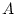and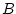are the same formula, but written in some different ways, then try proving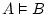or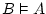.
• Take a truth and prove it. For instance: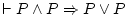.
• Take a lie, negate it, and try to prove that formula. Example: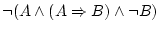. This method will make you practise reduction to the absurd.
• Convert some formula to its conjunctive normal form (so it is expressed like something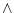something...something). Then you have several formulas which are all true at the same time: each of the conjunctands. You can select one of them and assert that when the original formula is true, then that conjunctand also is.
• Take several formulas at random, and suppose that all of them are true simultaneously. To do that, write their conjunction (oneotherother...). This big formula can be modified with the above methods to find some of its consequences. All this will be useful to practise natural deduction with several true formulas at the left part of the sequent.

Daniel Clemente Laboreo 2005-05-17